## 目录

### 简介

#### 欧姆定律Ohm’s law的向量形式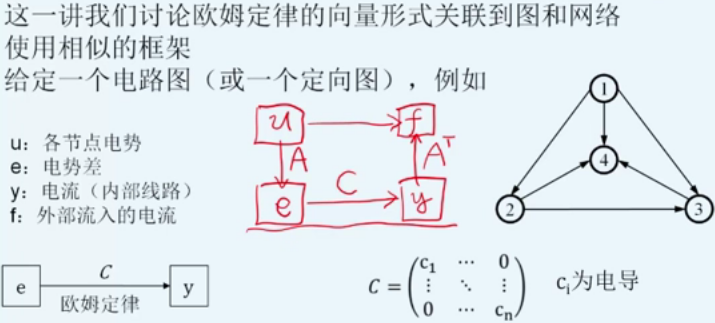### 图与矩阵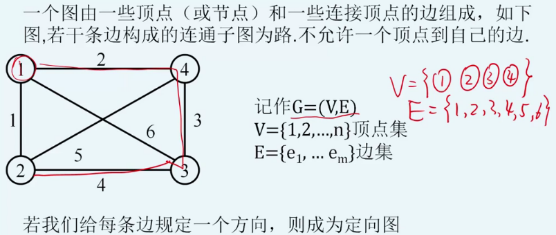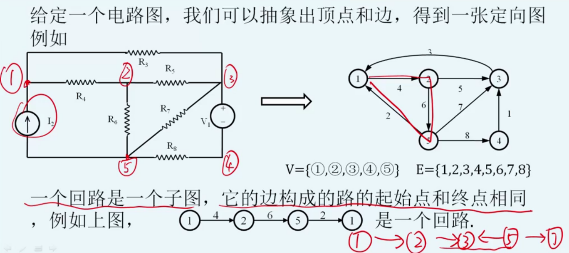#### 关联矩阵incidence matrix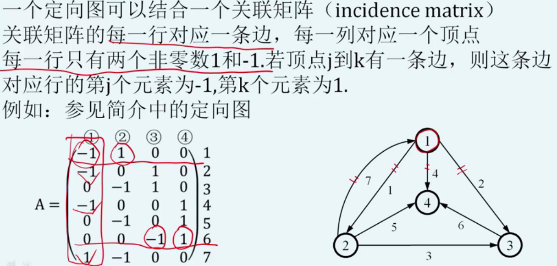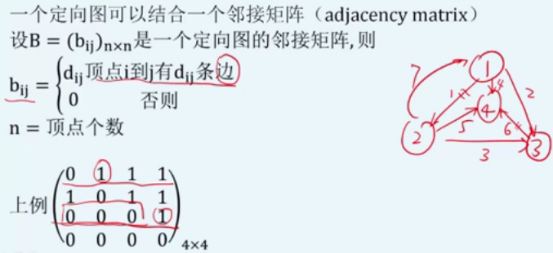#### 拉普拉斯矩阵laplacian matrix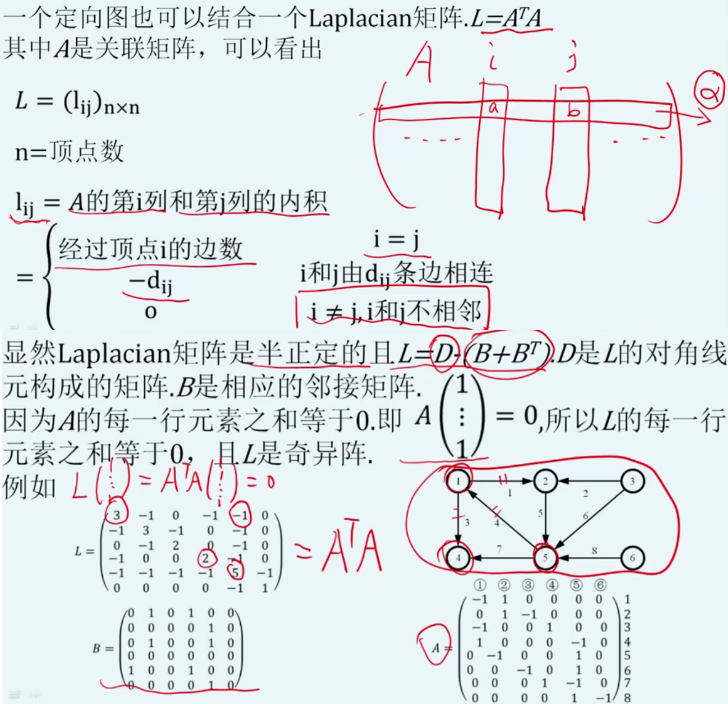### 网络和加权Laplacian矩阵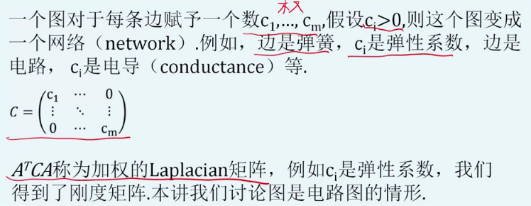#### 电路相关的物理定律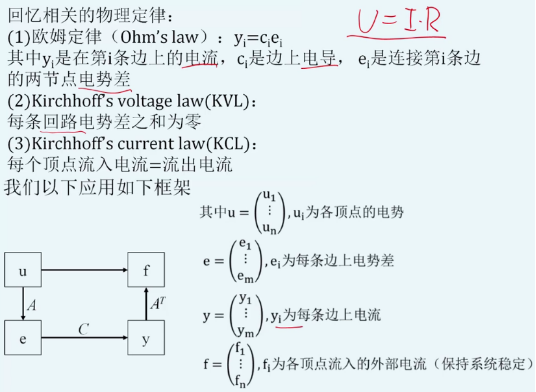#### 例子

##### 不接外部源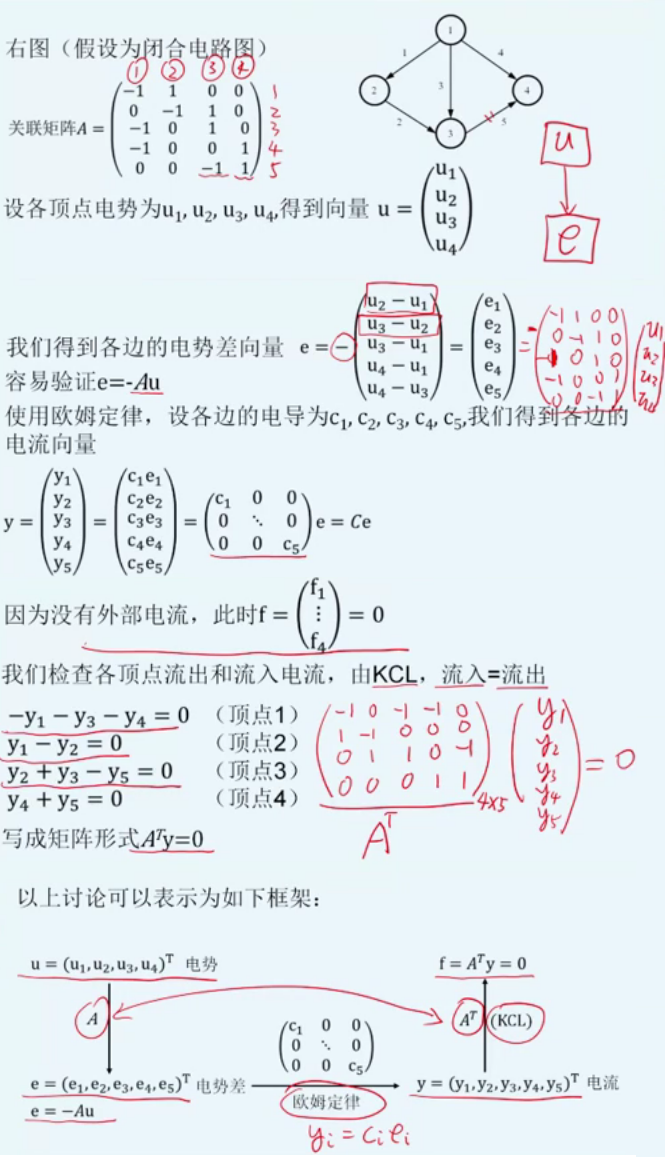#### 接外部源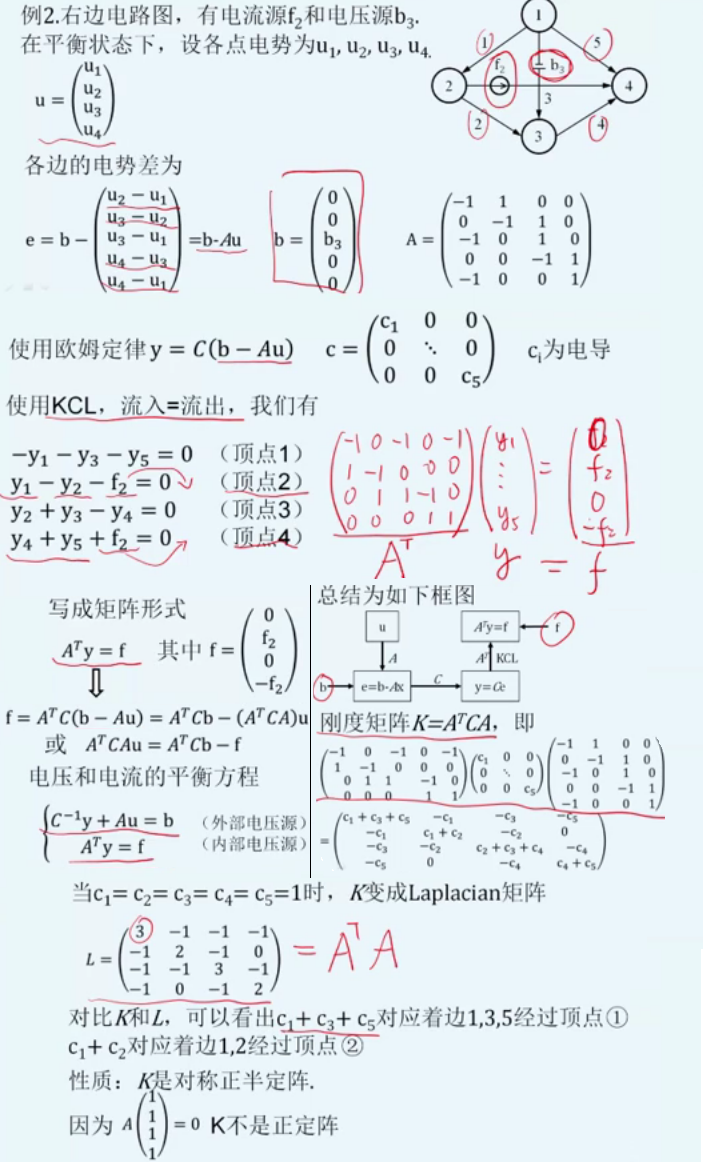#### 带权 K=ATCA K = A T C A $K=A^TCA$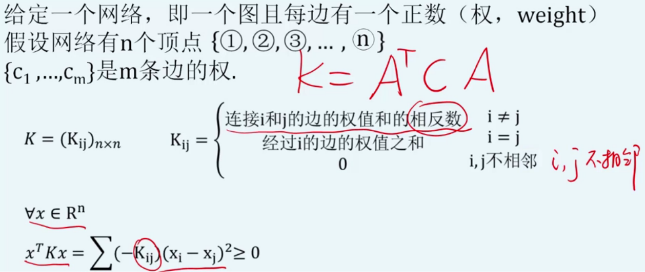### 关联矩阵的四个基本子空间

#### N(A)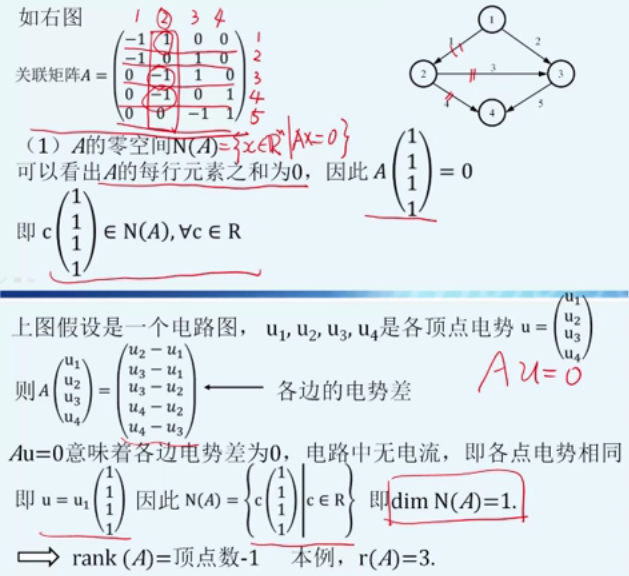#### C(A)

C(A) C ( A ) $C(A)$的定义得： C(A)={ Ax|xRn} C ( A ) = { A x | x ∈ R n } $C(A)=\{Ax|x\in R^n\}$ 。沿用前面使用的字母： u u $u$是各点电势， $e$$e$是各边电势差， Au=e A u = e $Au=e$ ，当 Au=e A u = e $Au=e$ 有解 eC(A) ⇔ e ∈ C ( A ) $\Leftrightarrow e \in C(A)$

1. 去证明： dim(C(A))=n1 d i m ( C ( A ) ) = n − 1 $dim(C(A))=n-1$ ，即 A A $A$ 的任意 $n-1$$n-1$个列向量是线性无关的。设 A=(a1,a2,...,an) A = ( a 1 , a 2 , . . . , a n ) $A=(a_1,a_2,\,...\,,a_n)$，不妨假设 a1,a2,...,an1 a 1 , a 2 , . . . , a n − 1 $a_1,a_2,\,...\,,a_{n-1}$线性相关，那么存在 c1,c2,...,cn1R c 1 , c 2 , . . . , c n − 1 ∈ R $c_1, c_2,\,...\,,c_{n-1} \in R$ 且不全为0满足： c1a1+c2a2+...+cn1an1+0an=0Ac1c2...cn10=0c1c2...cn10N(A), c 1 a 1 + c 2 a 2 + . . . + c n − 1 a n − 1 + 0 a n = 0 ⇒ A ( c 1 c 2 . . . c n − 1 0 ) = 0 ⇒ ( c 1 c 2 . . . c n − 1 0 ) ∈ N ( A ) , $c_1a_1+c_2a_2+...+c_{n-1}a_{n-1}+0a_n=0\Rightarrow A\begin{pmatrix}c_1\\c_2\\.\\.\\.\\c_{n-1}\\0\end{pmatrix}={0}\Rightarrow \begin{pmatrix}c_1\\c_2\\.\\.\\.\\c_{n-1}\\0\end{pmatrix}\in N(A),$但与 N(A)=c1...1cR N ( A ) = { c ( 1 . . . 1 ) | c ∈ R } $N(A)=\left\{c\begin{pmatrix}1\\.\\.\\.\\1\end{pmatrix} \Bigg| c\in R \right\}$ 矛盾，以此类推，得以证明 C(A) C ( A ) $C(A)$的维数是 n1 n − 1 $n-1$ ，即 A A $A$的任意 $n-1$$n-1$个列向量均可作为 C(A) C ( A ) $C(A)$的一组基。

2. 发现矩阵中对应的回路： eC(A) e ∈ C ( A ) $e\in C(A)$ 如下等式有解 Au=e11000101100110100011u1u2u3u4u5=e1e2e3e4e5u1+u2=e1u1+u3=e2u2+u3=e3u2+u4=e4u3+u4=e5{ e1e2+e3=0e3e4+e5=0 A u = e ⇒ ( − 1 1 0 0 − 1 0 1 0 0 − 1 1 0 0 − 1 0 1 0 0 − 1 1 ) ( u 1 u 2 u 3 u 4 u 5 ) = ( e 1 e 2 e 3 e 4 e 5 ) ⇒ { − u 1 + u 2 = e 1 − u 1 + u 3 = e 2 − u 2 + u 3 = e 3 − u 2 + u 4 = e 4 − u 3 + u 4 = e 5 ⇒ { e 1 − e 2 + e 3 = 0 e 3 − e 4 + e 5 = 0 $Au=e\Rightarrow \begin{pmatrix}-1&1&0&0\\-1&0&1&0\\0&-1&1&0\\0&-1&0&1\\0&0&-1&1\end{pmatrix}\begin{pmatrix}u_1\\u_2\\u_3\\u_4\\u_5 \end{pmatrix}=\begin{pmatrix}e_1\\e_2\\e_3\\e_4\\e_5 \end{pmatrix} \Rightarrow \begin{cases}-u_1+u_2=e_1\\-u_1+u_3=e_2\\-u_2+u_3=e_3\\-u_2+u_4=e_4\\-u_3+u_4=e_5\end{cases} \Rightarrow \begin{cases}e_1-e_2+e_3=0\\e_3-e_4+e_5=0\end{cases}$ ，即边1,2,3这3条边电势差之和为0，由图上可得边1,2,3恰好构成一个回路，边3,4,5也一样。这恰好是Kirchholff Voltage Law (KVL)。把这两个回路等式书写成矩阵形式 (1010110101)e1e2e3e4e5=0 ( 1 − 1 1 0 0 0 0 1 − 1 1 ) ( e 1 e 2 e 3 e 4 e 5 ) = 0 $\begin{pmatrix}1&-1&1&0&0\\0&0&1&-1&1 \end{pmatrix}\begin{pmatrix} e_1\\e_2\\e_3\\e_4\\e_5 \end{pmatrix}=0$ . 此时称矩阵 B=(1010110101) B = ( 1 − 1 1 0 0 0 0 1 − 1 1 ) $B =\begin{pmatrix}1&-1&1&0&0\\0&0&1&-1&1 \end{pmatrix}$回路矩阵，可以看到它的每一行代表一个回路且称为极小回路，每一列代表一条边。如果边的方向是逆时针方向则取为正号，否则取为负号。注意，此时 eN(B) e ∈ N ( B ) $e\in N(B)$

3. 此外， BA=(1010110101)11000101100110100011=(00000000) B A = ( 1 − 1 1 0 0 0 0 1 − 1 1 ) ( − 1 1 0 0 − 1 0 1 0 0 − 1 1 0 0 − 1 0 1 0 0 − 1 1 ) = ( 0 0 0 0 0 0 0 0 ) $BA=\begin{pmatrix}1&-1&1&0&0\\0&0&1&-1&1\end{pmatrix}\begin{pmatrix}-1&1&0&0\\-1&0&1&0\\0&-1&1&0\\0&-1&0&1\\0&0&-1&1\end{pmatrix}=\begin{pmatrix}0&0&0&0\\0&0&0&0\end{pmatrix}$ C(A)N(B) C ( A ) ⊆ N ( B ) $C(A) \subseteq N(B)$ dim(N(B))=3,dim(C(A))=3 d i m ( N ( B ) ) = 3 , d i m ( C ( A ) ) = 3 $dim(N(B))=3, dim(C(A))=3$，因此 C(A) C ( A ) $C(A)$就构成了 N(B) N ( B ) $N(B)$的基。从理意义角度理解： A A $A$矩阵执行的操作表示求解各边电势之差， $B$$B$各行刚好是回路，由 KVL K V L $KVL$定律得结果必为0.

#### N(AT) N ( A T ) $N(A^T)$

1. 由定义得： N(AT)={ yRm|ATy=0} N ( A T ) = { y ∈ R m | A T y = 0 } $N(A^T)=\{y\in R^m|A^Ty=0\}$。例子中，关联矩阵 A A $A$ 各行代表一条边，各列代表一个顶点。那么 ${A}^{T}$$A^T$ 的行代表顶点，列代表边。
ATy=011001010011001010011y1y2y3y4y5=00000y1y2=0y1y3y4=0y2+y3y5=0y4+y5=0 A T y = 0 ⇒ ( − 1 − 1 0 0 0 1 0 − 1 − 1 0 0 1 1 0 − 1 0 0 0 1 1 ) ( y 1 y 2 y 3 y 4 y 5 ) = ( 0 0 0 0 0 ) ⇒ { − y 1 − y 2 = 0 y 1 − y 3 − y 4 = 0 y 2 + y 3 − y 5 = 0 y 4 + y 5 = 0 $A^Ty=0\Rightarrow\begin{pmatrix}-1&-1&0&0&0\\1&0&-1&-1&0\\0&1&1&0&-1\\0&0&0&1&1\end{pmatrix}\begin{pmatrix}y_1\\y_2\\y_3\\y_4\\y_5 \end{pmatrix}=\begin{pmatrix}0\\0\\0\\0\\0\end{pmatrix} \Rightarrow \begin{cases}-y_1-y_2=0\\y_1-y_3-y_4=0\\y_2+y_3-y_5=0\\y_4+y_5=0\end{cases}$
物理意义解读： yi y i $y_i$是各第 i i $i$边上的电流，上述等式表明每一个顶点输入输出电流和为0，即Kichhoff Current Law (KCL)

2. ${A}^{T}y=0$$A^Ty=0$， 由前文得到：
BA=0ATBT=0ATBT=110010100110010100111110000111=00000000 B A = 0 ⇒ A T B T = 0 ⇒ A T B T = ( − 1 − 1 0 0 0 1 0 − 1 − 1 0 0 1 1 0 − 1 0 0 0 1 1 ) ( 1 0 − 1 0 1 1 0 − 1 0 1 ) = ( 0 0 0 0 0 0 0 0 ) $BA=0 \Rightarrow A^TB^T=0 \Rightarrow A^TB^T=\begin{pmatrix}-1&-1&0&0&0\\1&0&-1&-1&0\\0&1&1&0&-1\\0&0&0&1&1\end{pmatrix}\begin{pmatrix}1&0\\-1&0\\1&1\\0&-1\\0&1\end{pmatrix}=\begin{pmatrix}0&0\\0&0\\0&0\\0&0\end{pmatrix}$
因此， C(BT)N(AT) C ( B T ) ⊆ N ( A T ) $C(B^T) \subseteq N(A^T)$。由于 r(A)=C(A)=r=n1,N(AT)+C(A)=m,N(AT)=mr=53=2 r ( A ) = C ( A ) = r = n − 1 , N ( A T ) + C ( A ) = m , N ( A T ) = m − r = 5 − 3 = 2 $r(A)=C(A)=r=n-1, N(A^T)+C(A)=m, N(A^T)=m-r=5-3=2$， 由于 BT B T $B^T$的列向量线性无关，即 B B $B$的行向量代表回路，那么回路向量就是 $N\left({A}^{T}\right)$$N(A^T)$的一组基。

#### C(AT) C ( A T ) $C(A^T)$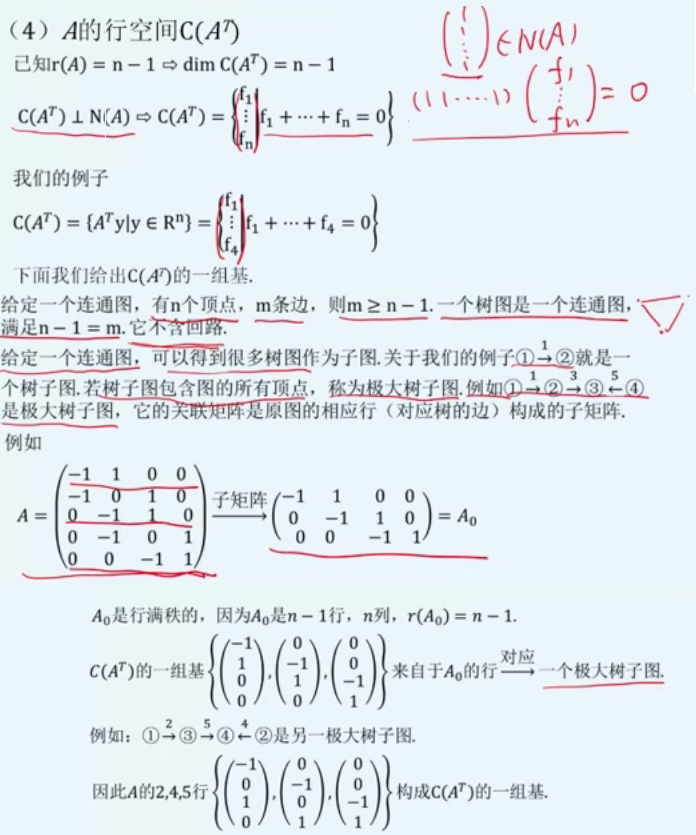#### 总结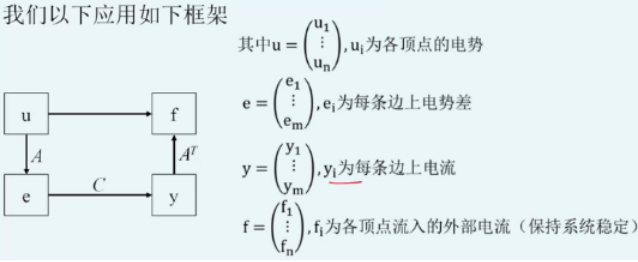• N(Am×n) N ( A m × n ) $N(A_{m\times n})$零空间 Au=0 A u = 0 $Au=0$ N(A)=c(1,1,...,1)Tn×1 N ( A ) = c ( 1 , 1 , . . . , 1 ) T n × 1 $N(A)=c{(1,1,\,...\,,1)^T}_{n\times 1}$ ；物理意义：各点电势相等，电势差为0。
• C(Am×n) C ( A m × n ) $C(A_{m\times n})$列空间 Au=e A u = e $Au=e$(上文用的是x, b)， A A $A$ 中任意 $n-1$$n-1$ 列构成了 C(A) C ( A ) $C(A)$ 的一组基；物理意义每个极小回路电势守恒，每个极小回路构成的极大回路电势依然守恒，诠释了KVL定律。
• N(AT) N ( A T ) $N(A^T)$左零空间 ATy=0 A T y = 0 $A^Ty=0$，回路向量构成了 N(AT) N ( A T ) $N(A^T)$ 的一组基；诠释了无外部电流源的KCL定律。
• C(AT) C ( A T ) $C(A^T)$行空间 ， ATy=f A T y = f $A^Ty=f$， 每个极大树子图对应关联矩阵的行向量（即边）构成了 C(AT) C ( A T ) $C(A^T)$ 的一组基；诠释了有外部电流源的KCL定律。

#### 注计

##### N(B)=C(A)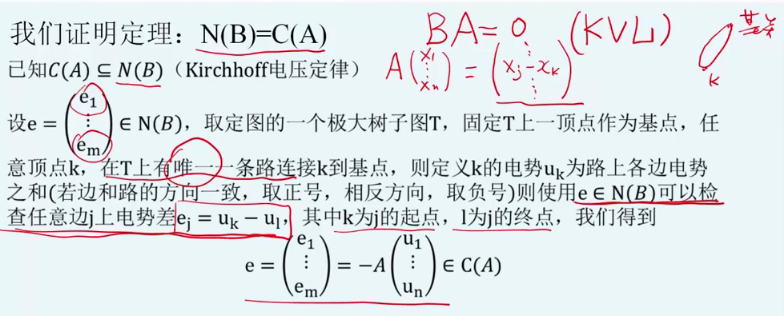B的零空间中的任何一个向量，它都要属于A的列空间 A A $A$的列空间中的每一个向量的特点，比如说 $A$$A$乘上一个 x1 x 1 $x_1$ xn x n $x_n$ x1 x 1 $x_1$ xn x n $x_n$ n n $n$个顶点的电势。 $A$$A$乘上这个向量得到的是各个边上的电势差，那么相应的 xjxk x j − x k $x_j-x_k$就是 j j $j$ $k$$k$两个顶点上的电势差，顶点连线， j j $j$ $k$$k$连线的边上的电势差。那么我们要想说明，N（B）中的向量属于C（A）那么我们只要说明任何一个向量属于B的零空间，它最后都能写成这样一种形式，就可以了。那么设 e e $e$属于 $N\left(B\right)$$N(B)$，那么我们可以取定这个连通图的一个极大树子图，然后在这个极大树子图 T T $T$上取一个顶点作为基点，那么任意的另外一个顶点 $K$$K$跟这个基点之间它们连线的路在 T T $T$上只有一条这样的路，因为 $T$$T$是一个树，它不可能有回路，所以在 T T $T$中有唯一的一条连接K到基点的路。定义K的电势：在这条路上各边的电势之和，各边的电势之和，我们这个 ${e}_{1}$$e_1$ em e m $e_m$呢，我们可以刻画各个边上的电势，那么我们可以看到 e e $e$属于 $N\left(B\right)$$N(B)$我们实际上可以检查出任意边上的电势差实际上是 ej e j $e_j$ uk u k $u_k$ u1 u 1 $u_1$，那么其中的这个 k k $k$呢为j的起点， $l$$l$ j j $j$的终点，最后我们就可以得到 $e=-Au$$e=-Au$，所以 e e $e$就属于 $C\left(A\right)$$C(A)$就是这个地方呢，我们要使用 e e $e$属于 $N\left(B\right)$$N(B)$，我们才能检查出：任意边上的这个电势差等于 uk u k $u_k$ ul u l $u_l$，就是要满足科尔霍夫电压定律。

#### 欧拉公式Euler’s formula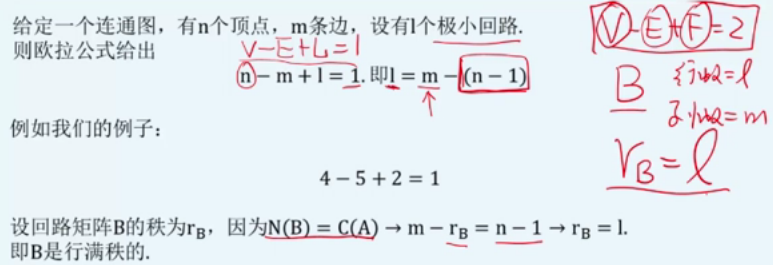### 网易之小易有一块n*n的棋盘，棋盘的每一个格子都为黑色或者白色，小易现在要用他喜欢的红色去涂画棋盘。小易会找出棋盘中某一列中拥有相同颜色的最大的区域去涂画_pomay的博客-程序员秘密

import java.util.Arrays;import java.util.Scanner;/** * 小易有一块n*n的棋盘，棋盘的每一个格子都为黑色或者白色，小易现在要用他喜欢的红色去涂画棋盘。小易会找出棋盘中某一列中拥有相同颜色的最大的区域去涂画， * 帮助小易算算他会涂画多少个棋格。 * * @author pomay * */public class Wang

### 车联网中如何应用大数据_车联网大数据_中琛源科技的博客-程序员秘密

车联网通过新一代信息通信技术，实现车与云平台、车与车、车与路、车与人、车内等全方位网络链接，主要实现了“三网融合”，即将车内网、车际网和车载移动互联网进行融合。车联网是利用传感技术感知车辆的状态信息，并借助无线通信网络与现代智能信息处理技术实现交通的智能化管理，以及交通信息服务的智能决策和车辆的智能化控制。　　1、车与云平台间的通信是指车辆通过卫星无线通信或移动蜂窝等无线通信技术实现与车联网服务平台的信息传输，接受平台下达的控制指令，实时共享车辆数据。　　2、车与车间的通信是指车辆与车辆之间实现

### python入门经典-终于明白经典python入门教程_编程大乐趣的博客-程序员秘密

Python是一种功能很强大的语言，对于零基础学习Python还是有难度的,但只要学习方法对，入门还是很快哒。下面介绍几种学习Python的方法。以下是小编为你整理的经典python入门教程首先是书籍，通过书籍学习，虽然速度会有些慢，但知识具体，可以掌握很多细节，一旦入门后，后面进步就很快了，下面介绍给大家一本书，是以前我学习Python时用的书，感觉还挺不错哒。然后就是借助网络学习，网上有很多视...

### 1~N中随机选三个数，求其最大的 最小公倍数。_陈梦夏的博客-程序员秘密

N的范围是1~10E6自己的想法是：N 的范围比较大，自己可以选出范围内最大的那个质数Z，然后再乘以范围最大的连个不同的数，就可以得出最大的 最小公倍数。错误第一个，这样的三个数直接相乘得到其最小公倍数，要求是这三个数必须两两互质，但是自己求出的三个数可能只满足 质数Z和其他两个数互质，但是其他的两个数不一定互质，所以求出的这个数不一定是 三个数的 最小公倍数。在把1~10E6范围内的质数打表